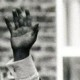## The maths of the spirograph… with the drawings

Well, I sat down and thought this was going to be easy, but it has taken me three hours to work the maths of a smaller inner wheel rolling around inside a large outer wheel: mainly because for the first two of those I neglected the basic insight that the inner wheel rolls in the opposite direction to its direction of travel (think of it this way – as a car wheel moves forward the point at the top of the wheel moves backwards – relative to the centre of the wheel).

And instead of using MetaPost I resorted to a spread sheet – though I might do a MetaPost drawing still.

Anyway – assume you have a big wheel of unit radius and a small wheel inside it of radius$\frac{1}{R}$.

At any given time the centre of this small wheel will be at cartesian co-ordinates (assuming the big wheel is centred on (0,0):

($cos(\theta)(1 -\frac{1}{R})$ ,$sin(\theta)(1 -\frac{1}{R})$) (1)

where$\theta$ is the angle of rotation of the small wheel relative to the centre of the big wheel.

But if the small wheel has moved through angle$\theta$ relative to the centre of the big wheel, then it will have itself rotated through the angle$R\theta$ – in the opposite direction to its rotation around the centre of the big wheel.

This means a fixed point on the surface of the small wheel will now be, compared to the centre of the smaller circle, at cartesian co-ordinates:

($\frac{cos(\theta - R\theta)}{R}$,$\frac{sin(\theta - R\theta)}{R}$) (2)

And we add (1) and (2) together to get the co-ordinates relative to the origin (ie the centre of the bigger circle).

Looking at the above it should be relatively obvious that if$R$ is an integer then the pattern will represent$R$ cusps – and not much less obvious is the fact that if$\frac{1}{R}$ can be expressed as a rational number then the pattern will repeat. But if$\frac{1}{R}$ cannot be expressed as a rational then it turns out there are a countably infinite number i.e.,$\aleph_0$, number of cusps. In a way this is just a graphical way of representing an irrational number – it is a number that cannot be made to divide up unity (the circle) into equal proportions.

So here are the pretty pictures:

Let$R = 2$ and we have a degenerate caseThen the 3 cusps of the ‘deltoid’:

### 2 responses to “The maths of the spirograph… with the drawings”

1.Thanks kindly for working this out. I have the dread suspicion that if I’d worked it out on my own I wouldn’t get the interior wheel orientation correct for an embarrassingly long time.

1.I had drawings in the book – so knew what I was after, but my figures did not look like the book at all, so had to work out why that was.